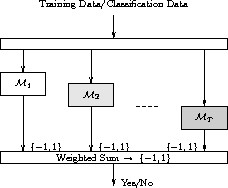DATA MINING
Desktop Survival Guide
by Graham Williams# BoostingThe Boosting meta-algorithm is an efficient, simple, and easy to understand model building strategy. The popular variant called AdaBoost (an abbreviation for Adaptive Boosting) has been described as the ``best off-the-shelf classifier in the world'' (attributed to Leo Breiman by (, p. 302)). Boosting algorithms build multiple models from a dataset, using some other model builders, such as a decision tree builder, that need not be a particularly good model builder. The basic idea of boosting is to associate a weight with each observation in the dataset. A series of models are built and the weights are increased (boosted) if a model incorrectly classifies the observation. The weights of such entities generally oscillate up and down from one model to the next. The final model is then an additive model constructed from the sequence of models, each model's output weighted by some score. There is little tuning required and little is assumed about the model builder used, except that it should be a relatively weak model builder! We note that boosting can fail to perform if there is insufficient data or if the weak models are overly complex. Boosting is also susceptible to noise.

Boosting is an example of an ensemble model builder.

Boosting builds a collection of models using a ``weak learner'' and thereby reduces misclassification error, bias, and variance (, ,). Boosting has been implemented in, for example, Refer[c50]C5.0. The term originates with ().

The algorithm is quite simple, beginning by building an initial model from the training dataset. Those entites in the training data which the model was unable to capture (i.e., the model mis-classifies those entites) have their weights boosted. A new model is then built with these boosted entities, which we might think of as the problematic entities in the training dataset. This model building followed by boosting is repeated until the specific generated model performs no better than random. The result is then a panel of models used to make a decision on new data by combining the ``expertise'' of each model in such a way that the more accurate experts carry more weight.

As a meta learner Boosting employs some other simple learning algorithm to build the models. The key is the use of a weak learning algorithm--essentially any weak learner can be used. A weak learning algorithm is one that is only somewhat better than random guessing in terms of error rates (i.e., the error rate is just below 50%). An example might be decision trees of depth 1 (i.e., decision stumps).

Subsections